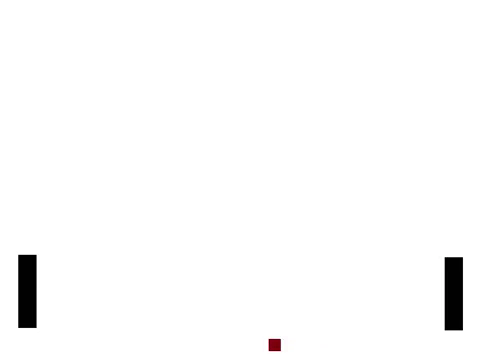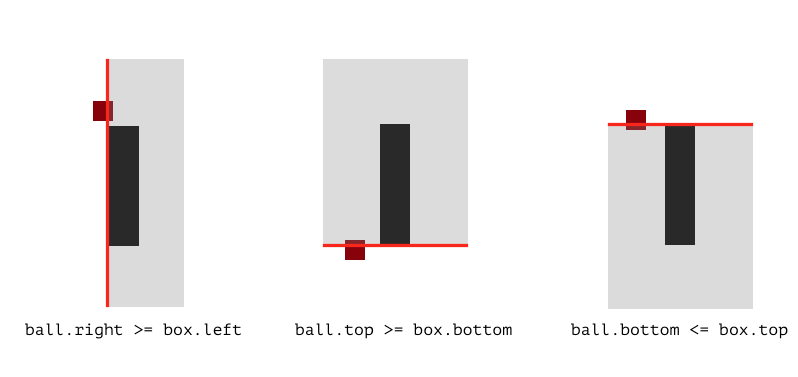👾🎉 Announcing Python Play (beta) & a pong game tutorial

# Python Play is the easiest way to get started coding games and graphics projects.

@amasad and the repl.it team asked me to help them make an easy way for new programmers to start making games and graphics projects. As a result, we made Python Play, a code library for Python loosely based on Scratch. For more information about Python Play, you can read the documentation here.

This is a tutorial showing how to use Python Play to make a game. To follow along with the tutorial, you can go to this repl and add code line-by-line.

The game we'll be making is a pong game:## How to make a pong game with Python Play

To make this game, first we need a box. Copying and pasting the code below will put a box on the screen:

``````import play # this should always be the first line in your program

box = play.new_box(color='black', x=0, y=0, width=30, height=120)

play.start_program() # this should always be the last line in your program``````

After you've copied that code, click the "Run" button. You should see a tall black box in the middle of the screen.

If you change any of the stuff after `new_box`, it will change what the box looks like and where it shows up on the screen. Change `x=0` to `x=100` and the box moves over to the right:

``box = play.new_box(color='black', x=350, y=0, width=30, height=120)``

(Click the Run button after every code change you make. Also make sure you still have the `import play` and `play.start_program()` lines of code in your program.)

Changing `x` changes the horizontal position and changing `y` changes the vertical position of the box. You can try playing with these numbers to see how they work. Don't forget you can do negative numbers i.e. `x=-100` (note the minus symbol in front).

Okay cool, a box is on the screen and we can change where it is. But how do we get it to do stuff? Change your code to look like this:

``````box = play.new_box(color='black', x=350, y=0, width=30, height=120)

@play.when_key_pressed('up')
async def do(key):
box.y += 10``````

Then try pressing the 'up' arrow on your keyboard. The box moves upward now!

The code above is saying "when the up arrow key is pressed, add `10` to the box's `y` position". Adding to the box's `y` position moves the box up on the screen. Can you guess how we could get the box to move down when the down arrow key is pressed?

Here's the full code for how you might do that:

``````box = play.new_box(color='black', x=350, y=0, width=30, height=120)

@play.when_key_pressed('up')
async def do(key):
box.y += 10

@play.when_key_pressed('down')
async def do(key):
box.y -= 10``````

(Remember that your program should still start with `import play` and end with `play.start_program()`.)

So now the box moves up and down on the screen when we press the arrow keys.

Now we need a ball. Add this line below the `new_box` line:

``ball = play.new_box(color='dark red', x=0, y=0, width=20, height=20)``

Now there's a ball but it's not moving.

To get it moving, here's the full code to put in your program:

``````ball = play.new_box(color='dark red', x=0, y=0, width=20, height=20)
ball.dx = 2
ball.dy = -1

# make the ball move
@play.repeat_forever
async def do():
ball.x += ball.dx
ball.y += ball.dy``````

This makes the ball move by changing its `x` and `y` position (repeating forever) by the horizontal speed `dx` and the vertical speed `dy`.

`ball.dx` and `ball.dy` are two variables we're making up to store the horizontal speed and vertical speed of the ball. The starting horizontal speed (`dx`) is 2 (to the right) and the vertical speed is -1 (down).

But the ball doesn't bounce off the paddle, it just goes right through. To fix that, we have to detect when the ball is right next to the paddle and reverse its direction. Add this code to your program:

``````# make the ball bounce off the player's paddle
@play.repeat_forever
async def do():
if (ball.right >= box.left) and (ball.top >= box.bottom) and (ball.bottom <= box.top) and (ball.left < box.left):
ball.dx = -2``````

Now the ball bounces off the paddle!

The code above checks three conditions which are best shown visually:If the ball is anywhere over the red line in the grey areas, then the condition written below it becomes `True`. If all three conditions are `True` at the same time, that means the ball hit the paddle and its speed should be reversed (-4) so it goes the other way. (`<=` means "less than or equal" and `>=` means "greater than or equal").

Here's the whole program at this point:

``````import play

box = play.new_box(color='black', x=350, y=0, width=30, height=120)

ball = play.new_box(color='dark red', x=0, y=0, width=20, height=20)
ball.dx = 2
ball.dy = -1

@play.when_key_pressed('up')
async def do(key):
box.y += 10

@play.when_key_pressed('down')
async def do(key):
box.y -= 10

@play.repeat_forever
async def do():
ball.x += ball.dx
ball.y += ball.dy

# make the ball bounce off the player's paddle
@play.repeat_forever
async def do():
if (ball.right >= box.left) and (ball.top >= box.bottom) and (ball.bottom <= box.top) and (ball.left < box.left):
ball.dx = -2

play.start_program()``````

There should be another player. Let's create another box! Add this code near where you put the code starting with `box = play.new_box`:

``````other_box = play.new_box(color='black', x=-350, y=0, width=30, height=120)
other_box.dy = 3``````

We're making the box have a vertical speed of 2 when it moves, but we haven't made it move yet.

To make the computer player follow the ball, we can add this code to our program:

``````# make the computer player follow the ball
@play.repeat_forever
async def do():
if ball.x < 0 and abs(ball.y-other_box.y) > other_box.dy:
if other_box.y < ball.y:
other_box.y += other_box.dy
elif other_box.y > ball.y:
other_box.y -= other_box.dy``````

Now when the ball is on the left side of the screen, the computer player will move toward the ball! We add to the box's `y` if the box is below the ball, otherwise we subtract from the box's `y` if it's above the ball.

But oops, the ball doesn't bounce off the computer player's paddle (`other_box`). Let's make it do that by adding this code:

``````# make the ball bounce off the computer player's paddle
@play.repeat_forever
async def do():
if (ball.left <= other_box.right) and (ball.top >= other_box.bottom) and (ball.bottom <= other_box.top) and (ball.right > other_box.right):
other_box.dy = play.random_number(1, 4)
ball.dx = 2``````

This code works just like the collision code from above but in reverse for the left paddle. Also when the ball hits the paddle we change the paddle's speed to a random number between 1 and 4 so the paddle will move either slower or faster.

But oops again, now if we get the ball to bounce off the computer player's paddle, it doesn't bounce off the walls. To make the ball bounce off the walls, we add this code that checks that the ball is lower than the top of the screen and higher than the bottom of the screen:

``````# make ball bounce off bottom and top walls
@play.repeat_forever
async def do():
if ball.bottom <= play.screen.bottom:
ball.dy = 1
elif ball.top >= play.screen.top:
ball.dy = -1``````

If the ball hits either the top or the bottom of the screen, the code above will reverse its speed so it bounces.

And that's it! A simple pong game in about 50 lines of code!

## The final code

Here's all the code in the tutorial in one place:

``````import play

box = play.new_box(color='black', x=350, y=0, width=30, height=120)

other_box = play.new_box(color='black', x=-350, y=0, width=30, height=120)
other_box.dy = 3

ball = play.new_box(color='dark red', x=0, y=0, width=20, height=20)
ball.dx = 2
ball.dy = -1

@play.when_key_pressed('up')
async def do(key):
box.y += 10

@play.when_key_pressed('down')
async def do(key):
box.y -= 10

# make the ball move
@play.repeat_forever
async def do():
ball.x += ball.dx
ball.y += ball.dy

# make the ball bounce off the player's paddle
@play.repeat_forever
async def do():
if (ball.right >= box.left) and (ball.top >= box.bottom) and (ball.bottom <= box.top) and (ball.left < box.left):
ball.dx = -2

# make the computer player follow the ball
@play.repeat_forever
async def do():
if ball.x < 0 and abs(ball.y-other_box.y) > other_box.dy:
if other_box.y < ball.y:
other_box.y += other_box.dy
elif other_box.y > ball.y:
other_box.y -= other_box.dy

# make the ball bounce off the computer player's paddle
@play.repeat_forever
async def do():
if (ball.left <= other_box.right) and (ball.top >= other_box.bottom) and (ball.bottom <= other_box.top) and (ball.right > other_box.right):
other_box.dy = play.random_number(1, 4)
ball.dx = 2

# make ball bounce off bottom and top walls
@play.repeat_forever
async def do():
if ball.bottom <= play.screen.bottom:
ball.dy = 1
elif ball.top >= play.screen.top:
ball.dy = -1

play.start_program()``````

And here's a link to a repl with the code above.

## More Challenges

This game is pretty simple. Can you think of other things to add to make it more fun? Here are some suggestions for things to try:

• Can you make the paddles change colors when the ball hits them?
• How would you keep track of and show scores in the game? (Hint: look up the `play.new_text()` function.)
• Did you find any glitches in the game? How would you try to fix those glitches?
• How would you make the ball change speed differently depending on where it hits on the paddle?
• Could you add multiple balls to the game?

What else would you add to make the game more fun?

# Python Play

Thanks for reading this tutorial! If you make anything with Python Play, please post it in the comments!

Python Play is currently in beta, which means some things may not work quite right. If you find a problem (usually called a "bug"), please send us a link to the repl where you found that bug.

To find out more about all the things you can do with Python Play, read the documentation here!

Look for more Python Play features coming soon! Try it out and let us know what you think!

You are viewing a single comment. View All
mkhoi (309)

Finally i can do a cooler game than a text-based adventure with ease